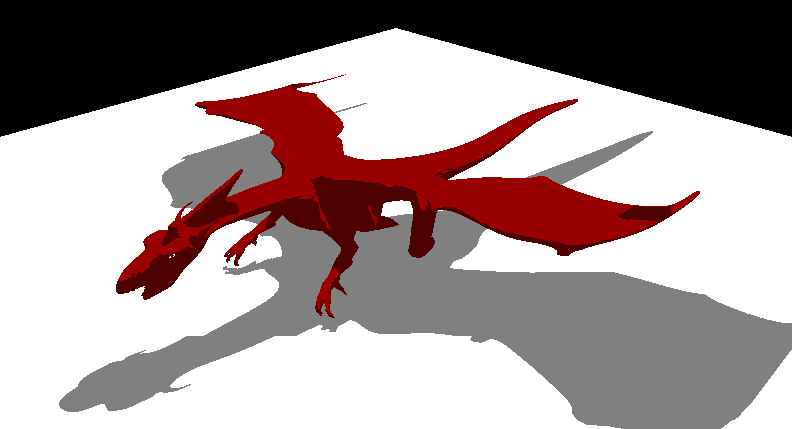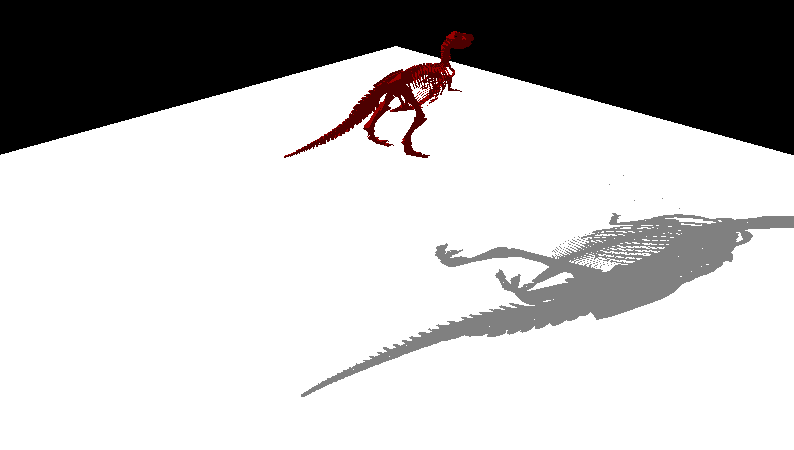## Published: July 2011

Here I describe simple algorithm to calculate silhouettes given a 3d model and a light position (or direction) quite quickly. The algorithm is compared to the classical approach which is the typical implementation for simple programs. This is the algorithm which uses Toy Wars and provides a very fast calculation which makes a significant speedup on PSP platform.

There's also a connectivity based algorithm which I've been designing last days and which I expect to integrate on Toy Wars too.

# Classical approach

The classical approach is well explained here: The Theory of Stencil Shadow Volumes

In brief it consists on keeping a list of edges candidates to silhouette and updating it by adding candidates and removing the ones already in the list. This approach is completely brute-force. In the worse case the algorithm takes something near O(n²). Also, depending on how it is implemented (using linked list, arrays, etc...) it will make lots of allocations and deallocations, which will lead to a fragmented heap and slow performance.

On the other hand this approach is very robust for almost any kind of 3d geometry. It doesn't matter if the mesh is convex, it will find a good silhouette or at least a good one for casting a stencil shadow.

# Fast implementation

This implementation is based on the same principle of the classical one. The idea is to replace the list of candidate edges with a hash table. The table has as many rows as possible number of vertices. The column number is a little number (such as 8, 16, 32...) which is calculated as the maximum number of triangles sharing the same vertex. The complexity of the algorithm is O(n*numcols), so using a lower number of columns is good, but if the number is too low the algorithm will fail. This is its biggest problem: the number of triangles sharing a vertex is limited. On typical game usage this can be avoided by manually tweaking the number based on the game needs.

It works by iterating all the light facing triangles and doing for their 3 edges:

1. Naming v1 the first vertex of the edge and v2 the second one, access the table row number v2.
2. Iterate for every column looking for the value v1. If found, mark the cell as empty and continue to the next edge (step 1).
3. In case it's not found access the row v1 and look for an empty cell to insert value v2.

The table needs an empty cell mark. I use ~0 for marking an empty cell, so I memset all the table with binary ones. The idea is to represent each edge by the row number (the first vertex) and the cell value (second vertex). So each row needs as many columns as possible number of edges having the same first vertex and different second vertices. At the end we'll have in the table only the edges candidates to silhouette.

This implementation is very fast and robust. Using the original algorithm provides us a robust silhouette in front of other algorithms based on walking trough the silhouette. The implementation can be very efficient as it's based on table lookups (that's why I use a power of two number for the column number) and fixed size loops (which can be unrolled very efficiently by the compiler). Using 16-bit indices (common on lots of applications) gives us an extra memory save and can help to fit the table rows in cache blocks. This is particularly good, so we can use memalign to align the table to cache block size boundary.

# Connectivity table

In order to take advantage of the static data this new approach precalculates a connectivity table, which maps each edge to a triangle, which also shares the edge but reversed.

The calculation is slow (O(n²) where n is the number of edges) and the table isn't as little as we could desire, but it's smaller than the hash algorithm's in some cases. The big advantage is that the silhouette determination takes O(3*n) where n is the number of faces. So as can be easily seen the algorithm is quite fast as compared to the previous methods.

The algorithm does the following steps for each possible edge (number of triangles * 3).

1. Test if the triangle is lit (light facing).
2. If true, look up the neighbor triangle and check if it's unlit (not facing light)
3. If true, emit that edge as silhouette edge.

The algorithm makes redundant tests for lit/unlit triangle, as opposed to the previous ones that were triangle-based. A lookup table or a hash table could be used to avoid recalculations, but as we know the test will be performed three times in the worst case it's not worth the effort, there won't be any gain.

# Some benchmarking

I ran some tests with a couple of 3d models and I got some timings on my laptop:

Classic Fast (64) Fast (8) Connectivity table
Dino 9000ms 160ms 49ms 31ms
Dragon 2100ms 270ms 44ms 13ms

The number shown inside the parenthesis is the number of columns of the hash table. It's very clear that the maximum gain is produced by the dino model. This model has a very large silhouette (with lots of edges) which leads to a very big list in the classical approach. The other algorithms behave quite well, but there's not much difference.

In the case of the dragon the classic is faster because of the smaller silhouette and the other algorithms behave faster. The Fast implementation behaves slower (as seen in the 64 case) but the connectivity it's very fast. This can be explained by the light position, which is hanging above the dragon and leads to a lot of triangles back-facing the light. This speeds a lot the calculation, because if a triangle backfaces the light no further calculation needs to be done, so the algorithm skips a lot of calculations in those cases.

# Conclusions

As seen before, the connectivity table algorithm is very fast in comparison to the other implementations. But we haven't analyzed other factors which can be important when using the algorithms in the "real world". Those factors are the memory usage, which can be divided into temporal memory (used by the classic and fast approach), the permanent memory usage (used by the connectivity table) and the precomputation time (which is significant in the connectivity table calculation).

All the algorithms use the same principle of silhouette calculation, so they're robust and deterministic (so they don't depend on the previous light or shadow data). Also they are very simple and easy to optimize, specially the table-based ones.

Having the possibility to precalculate the connectivity table and store it with the 3d model on disk I'd go for this one, and leave the hash based algorithm for the ocasions where we can't precalculate the connectivity table (such as on the fly geometry generation)

# Models usedDragon. Num faces: 63744. Num verts: 32859.Dino. Num faces: 28766. Num verts: 60725